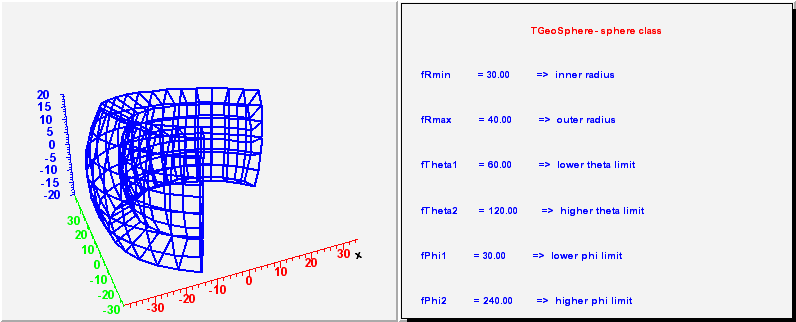library: libGeom#include "TGeoSphere.h"

# TGeoSphere

class description - source file - inheritance tree (.pdf)

## class TGeoSphere : public TGeoBBox

Inheritance Chart:
 TObject
<-
TNamed
<-
TGeoShape
<-
TGeoBBox
<-
TGeoSphere
```
public:
TGeoSphere()
TGeoSphere(Double_t rmin, Double_t rmax, Double_t theta1 = 0, Double_t theta2 = 180, Double_t phi1 = 0, Double_t phi2 = 360)
TGeoSphere(const char* name, Double_t rmin, Double_t rmax, Double_t theta1 = 0, Double_t theta2 = 180, Double_t phi1 = 0, Double_t phi2 = 360)
TGeoSphere(Double_t* param, Int_t nparam = 6)
TGeoSphere(const TGeoSphere&)
virtual ~TGeoSphere()
static TClass* Class()
virtual void ComputeBBox()
virtual void ComputeNormal(Double_t* point, Double_t* dir, Double_t* norm)
virtual Bool_t Contains(Double_t* point) const
virtual Int_t DistancetoPrimitive(Int_t px, Int_t py)
virtual Double_t DistFromInside(Double_t* point, Double_t* dir, Int_t iact = 1, Double_t step = TGeoShape::Big(), Double_t* safe = 0) const
virtual Double_t DistFromOutside(Double_t* point, Double_t* dir, Int_t iact = 1, Double_t step = TGeoShape::Big(), Double_t* safe = 0) const
Double_t DistToSphere(Double_t* point, Double_t* dir, Double_t rsph, Bool_t check = kTRUE, Bool_t firstcross = kTRUE) const
virtual TGeoVolume* Divide(TGeoVolume* voldiv, const char* divname, Int_t iaxis, Int_t ndiv, Double_t start, Double_t step)
virtual const char* GetAxisName(Int_t iaxis) const
virtual Double_t GetAxisRange(Int_t iaxis, Double_t& xlo, Double_t& xhi) const
virtual void GetBoundingCylinder(Double_t* param) const
virtual const TBuffer3D& GetBuffer3D(Int_t reqSections, Bool_t localFrame) const
virtual Int_t GetByteCount() const
virtual TGeoShape* GetMakeRuntimeShape(TGeoShape*, TGeoMatrix*) const
virtual Int_t GetNmeshVertices() const
Int_t GetNumberOfDivisions() const
Int_t GetNz() const
Double_t GetPhi1() const
Double_t GetPhi2() const
virtual Double_t GetRmax() const
virtual Double_t GetRmin() const
Double_t GetTheta1() const
Double_t GetTheta2() const
virtual void InspectShape() const
virtual TClass* IsA() const
virtual Bool_t IsCylType() const
Bool_t IsPointInside(Double_t* point, Bool_t checkR = kTRUE, Bool_t checkTh = kTRUE, Bool_t checkPh = kTRUE) const
virtual TBuffer3D* MakeBuffer3D() const
TGeoSphere& operator=(const TGeoSphere&)
virtual Double_t Safety(Double_t* point, Bool_t in = kTRUE) const
virtual void SavePrimitive(ofstream& out, Option_t* option)
virtual void SetDimensions(Double_t* param)
virtual void SetNumberOfDivisions(Int_t p)
virtual void SetPoints(Double_t* points) const
virtual void SetPoints(Float_t* points) const
virtual void SetSegsAndPols(TBuffer3D& buff) const
void SetSphDimensions(Double_t rmin, Double_t rmax, Double_t theta1, Double_t theta2, Double_t phi1, Double_t phi2)
virtual void ShowMembers(TMemberInspector& insp, char* parent)
virtual void Sizeof3D() const
virtual void Streamer(TBuffer& b)
void StreamerNVirtual(TBuffer& b)
```

### Data Members

```
protected:
Int_t fNz      number of z planes for drawing
Int_t fNseg    number of segments for drawing
Double_t fTheta1  lower theta limit
Double_t fTheta2  higher theta limit
Double_t fPhi1    lower phi limit
Double_t fPhi2    higher phi limit
```

## Class Description

``` TGeoSphere - spherical shell class. It takes 6 parameters :
- inner and outer radius Rmin, Rmax
- the theta limits Tmin, Tmax
- the phi limits Pmin, Pmax (the sector in phi is considered
starting from Pmin to Pmax counter-clockwise

_____________________________________________________________________________
```
/**/

TGeoSphere()
``` Default constructor
```

TGeoSphere(Double_t rmin, Double_t rmax, Double_t theta1, Double_t theta2, Double_t phi1, Double_t phi2) :TGeoBBox(0, 0, 0)
``` Default constructor specifying minimum and maximum radius
```

TGeoSphere(const char *name, Double_t rmin, Double_t rmax, Double_t theta1, Double_t theta2, Double_t phi1, Double_t phi2) :TGeoBBox(name, 0, 0, 0)
``` Default constructor specifying minimum and maximum radius
```

TGeoSphere(Double_t *param, Int_t /*nparam*/) :TGeoBBox(0, 0, 0)
``` Default constructor specifying minimum and maximum radius
param = Rmin
param = Rmax
```

~TGeoSphere()
``` destructor
```

void ComputeBBox()
``` compute bounding box of the sphere
Double_t xmin, xmax, ymin, ymax, zmin, zmax;
```

void ComputeNormal(Double_t *point, Double_t *dir, Double_t *norm)
``` Compute normal to closest surface from POINT.
```

Bool_t IsPointInside(Double_t *point, Bool_t checkR, Bool_t checkTh, Bool_t checkPh) const

Bool_t Contains(Double_t *point) const
``` test if point is inside this sphere
check Rmin<=R<=Rmax
```

Int_t DistancetoPrimitive(Int_t px, Int_t py)
``` compute closest distance from point px,py to each corner
```

Double_t DistFromOutside(Double_t *point, Double_t *dir, Int_t iact, Double_t step, Double_t *safe) const
``` compute distance from outside point to surface of the sphere
```

Double_t DistFromInside(Double_t *point, Double_t *dir, Int_t iact, Double_t step, Double_t *safe) const
``` compute distance from inside point to surface of the sphere
```

Double_t DistToSphere(Double_t *point, Double_t *dir, Double_t rsph, Bool_t check, Bool_t firstcross) const
``` compute distance to sphere of radius rsph. Direction has to be a unit vector
```

TGeoVolume* Divide(TGeoVolume * /*voldiv*/, const char * /*divname*/, Int_t /*iaxis*/, Int_t /*ndiv*/, Double_t /*start*/, Double_t /*step*/)
``` Divide all range of iaxis in range/step cells
```

const char* GetAxisName(Int_t iaxis) const
``` Returns name of axis IAXIS.
```

Double_t GetAxisRange(Int_t iaxis, Double_t &xlo, Double_t &xhi) const
``` Get range of shape for a given axis.
```

void GetBoundingCylinder(Double_t *param) const
```--- Fill vector param with the bounding cylinder parameters. The order
is the following : Rmin, Rmax, Phi1, Phi2
```

void InspectShape() const
``` print shape parameters
```

TBuffer3D* MakeBuffer3D() const
``` Creates a TBuffer3D describing *this* shape.
Coordinates are in local reference frame.
```

void SetSegsAndPols(TBuffer3D & buff) const
``` Fill TBuffer3D structure for segments and polygons.
```

Double_t Safety(Double_t *point, Bool_t in) const
``` computes the closest distance from given point to this shape, according
to option. The matching point on the shape is stored in spoint.
```

void SavePrimitive(ofstream &out, Option_t * /*option*/)
``` Save a primitive as a C++ statement(s) on output stream "out".
```

void SetSphDimensions(Double_t rmin, Double_t rmax, Double_t theta1, Double_t theta2, Double_t phi1, Double_t phi2)

void SetDimensions(Double_t *param)

void SetNumberOfDivisions(Int_t p)

void SetPoints(Double_t *points) const
``` create sphere mesh points
```

void SetPoints(Float_t *points) const
``` create sphere mesh points
```

Int_t GetNmeshVertices() const
``` Return number of vertices of the mesh representation
```

void Sizeof3D() const
```/// obsolete - to be removed
```

const TBuffer3D& GetBuffer3D(Int_t reqSections, Bool_t localFrame) const

### Inline Functions

```              Int_t GetByteCount() const
TGeoShape* GetMakeRuntimeShape(TGeoShape*, TGeoMatrix*) const
Int_t GetNumberOfDivisions() const
Int_t GetNz() const
Double_t GetRmin() const
Double_t GetRmax() const
Double_t GetTheta1() const
Double_t GetTheta2() const
Double_t GetPhi1() const
Double_t GetPhi2() const
Bool_t IsCylType() const
TClass* Class()
TClass* IsA() const
void ShowMembers(TMemberInspector& insp, char* parent)
void Streamer(TBuffer& b)
void StreamerNVirtual(TBuffer& b)
TGeoSphere TGeoSphere(const TGeoSphere&)
TGeoSphere& operator=(const TGeoSphere&)
```

Author: Andrei Gheata 31/01/02
Last update: root/geom:\$Name: \$:\$Id: TGeoSphere.cxx,v 1.45 2005/09/04 15:12:08 brun Exp \$